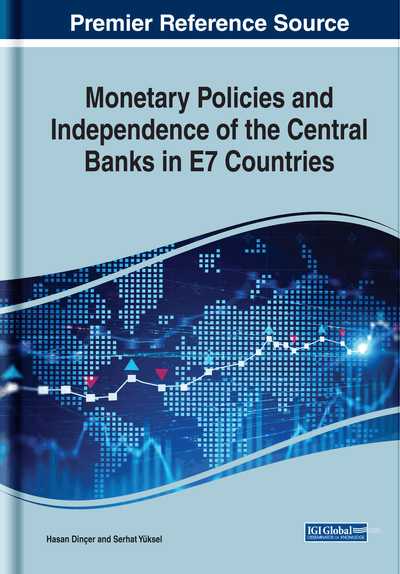# Fuzzy Multicriteria Decision Making Methodologies Used in the Book

DOI: 10.4018/978-1-7998-1643-0.ch005
Available
\$37.50
No Current Special Offers

## Abstract

Using an appropriate methodology is crucial in the analysis. Therefore, the suitable model should be selected according to the type of the evaluation. Otherwise, there is a risk of having inappropriate results. Because of this situation, recommendations can be problematic. In this book, three different analyses are performed. In two of them, fuzzy DEMATEL, fuzzy TOPSIS, and fuzzy VIKOR approaches are taken into consideration. In this chapter, these three methods are explained. In this framework, some studies, which used these methods, are explained.
Chapter Preview
Top

## Fuzzy Dematel

The word DEMATEL refers to the expression of “The Decision Making Trial and Evaluation Laboratory”. As it can be understood from this expression, this method is mainly used to make decisions under the complex environment. This model was developed by Gabus and Fontela in Genova research center. Another important benefit of this method is that it can be considered in order to understand the causal relationship between the criteria (Vinodh et al., 2016; Dinçer, 2018). In addition to them, impact relationship analysis can be performed by using this model. This situation provides a significant benefit to DEMATEL approach in comparison with the similar models, such as AHP and ANP (Dinçer et al., 2018; Gorener et al., 2013; Dinçer and Gorener, 2011; Dinçer, 2015). In summary, complex problems can be solved much easier with the help of this methodology (Lin et al., 2018; Dinçer and Hacıoğlu, 2017). There are mainly six different steps in DEMATEL model. The details of these steps are given below.

In the first step, the main purpose is determined so as to solve the problem. Moreover, in the second step, different criteria are generated in order to make evaluation. Furthermore, fuzzy linguistic scale is defined. Therefore, it can be possible to solve problems in case of uncertainty because there is human assessment process. In the development process of this scale, five different aspects are taken into consideration which are “No”, “Low”, “Medium”, “High”, “Very High”.

Additionally, the third step includes the calculation of the initial direct-relation fuzzy matrix. In order to reach this objective, the decision makers’ evaluations are taken into consideration for each dimension and criterion. In addition to these issues, triangular fuzzy numbers are also considered for the development of this matrix. The calculation process of the initial direct-relation fuzzy matrix () is demonstrated on the equations (1) and (2).

(1)

## Complete Chapter List

Search this Book:
Reset